# Math Challenge - August 2021

• Challenge
• Featured
Mentor
Summary: countability, topological vector spaces, continuity of linear maps, polynomials, finite fields, function theory, calculus

1. Let ##(X,\rho)## be a metric space, and suppose that there exists a sequence ##(f_i)_i## of real-valued continuous functions on ##X## with the property that a Cauchy sequence ##(x_n)_n## is convergent whenever each of the sequences ##(f_i(x_n))_i## is bounded. Then ##X## can be remetrized (with equivalent metrics) so as to be complete.

2. Let ##X=C([0,1])## be the topological space of real-valued continuous functions,
$$\rho(f,g):=\operatorname{sup}_{x\in [0,1]}|f(x)-g(x)|$$
the uniform metric induced by the ##L^\infty ## norm,
$$\sigma (f,g):=\int_0^1 |f(x)-g(x)|\,dx$$
the ##L^1## induced metric, and for n∈N
$$E_n:=\left\{\left. f\in X\,\right|\,\exists \,x\in \left[0,1-1/n\right]\,\forall \,h\in (0,1-x)\, : \,|f(x+h)-f(x)|\leq nh\right\}.$$
Show that
1. ##(X,\rho)## is complete.
2. ##(X,\rho) \not\cong (X,\sigma)## is not complete.
3. ##E_n\subseteq (X,\rho)## is closed.

3. (solved by @QuantumSpace ) Show that the set of real algebraic numbers is infinite, and denumerable.

4. A topology ##\mathcal{T}## on a vector space ##L## over a non-discrete topological field ##K## defines a topological vector space, i.e. addition and scalar multiplication are continuous, if and only if ##\mathcal{T}## is translation-invariant (all mappings ##x\mapsto x+x_0## are homeomorphisms) and possesses a ##0-##neighborhood base ##\mathcal{B}## with the following properties:
• For each ##V\in \mathcal{B},## there exists ##U\in \mathcal{B}## such that ##U+U\subseteq V.##
• Every ##V\in \mathcal{B}## is radial (i.e. there exists a ##\lambda_0 \in K## such that whenever ##|\lambda|\geq |\lambda_0| ## we have ##F\subseteq \lambda V## for each finite subset ##F\subseteq L##) and circled (##\lambda V\subseteq V## whenever ##|\lambda |\leq 1##).
• There exists ##\lambda \in K,\,0<|\lambda |<1,## such that ##V\in \mathcal{B}## implies ##\lambda V\in \mathcal{B}.##
If ##K## is an Archimedean valuated field, e.g. ##K\in \{\mathbb{R},\mathbb{C}\},## then the last condition is dispensable.

5. Let ##L\stackrel{\mu}{\longrightarrow }M## be locally convex topological vector spaces, ##\mathcal{P}## a family of semi-norms (##\|\alpha x\|_p = |\alpha |\cdot \|y\|_p## and ##\|x+y\|_p\leq \|x\|_p+\|y\|_p##) generating the topology of ##L## and ##\mu## algebraically homomorph, i.e. linear. Then ##\mu## is continuous if and only if for each continuous semi-norm ##q## on ##M,## there exists a finite subset ##\{p_j\,|\,j=1,\ldots,n\}\subseteq \mathcal{P}## and a number ##c>0## such that ##\|\mu(x)\|_q < c\cdot \sup_j p_j(x)## for all ##x\in L.##

6. Let ##\mathbb{F}_q## be a finite field of characteristic ##p.## Show that it's multiplicative group ##\mathbb{F}^*_q=\mathbb{F}_q-\{0\}## is cyclic.

7. Let ##\mathbb{F}_q## be a finite field of characteristic ##p## and ##f_\alpha \in \mathbb{F}_q[X_1,\ldots,X_n]## polynomials such that ##\sum_\alpha \deg f_\alpha <n,## and ##V\subseteq \mathbb{F}_q^n## be the set of their common zeros. Then
$$p\,|\,\operatorname{card}(V)$$

8. (solved by @julian ) A linear fractional transformation ##S\, : \,\mathbb{C}_\infty \longrightarrow \mathbb{C}_\infty ## defined by ##S(z)=\dfrac{az+b}{cz+d}## is called a Möbius transformation if ##ad-bc\neq 0##. Here ##S(\infty )=a/c## and ##S(-d/c)=\infty .## Show that Möbius transformations form a group by composition, and that there is a unique Möbius transformation ##S(z)## which takes ##(z_1,z_2,z_3)## to ##(1,0,\infty ).## Which one?

9. Let ##G## be a connected open set and let ##f:G\longrightarrow \mathbb{C}## be an analytic function. Show that the following statements are equivalent:
1. ##f\equiv 0##
2. There is a point ##a\in G## such that ##f^{(n)}(a)=0## for each ##n\geq 0.##
3. ##\{z\in G\,|\,f(z)=0\}## has a limit point in ##G.##

10. Suppose ##f## and ##g## are meromorphic in a neighborhood of ##\overline{B}(a;R)## with no zeros (##Z##) or poles (##P##) on the circle ##\gamma =\{z\in \mathbb{C}\,|\,|z-a|=R\} ##. If ##Z_f,Z_g,P_f,P_g## are the numbers of zeros, resp. poles, of ##f## and ##g## inside ##\gamma ## counted according to their multiplicities and if
$$|f(z)+g(z)|<|f(z)|+|g(z)|$$
on ##\gamma ,## then
$$Z_f-P_f=Z_g-P_g\;.$$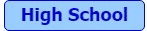High Schoolers only

11.
(solved by @Not anonymous ) A gardener holds a water hose horizontally and wants to water a bed ##6\,\rm m## away. The water exits the hose at a speed of ##8\,\rm m/s\,.## Calculate the minimum height the gardener needs to hold the hose for the water to reach the bed, the speed at which the water droplets hit the bed, and the angle at which the water droplets hit the bed.

12. (solved by @Not anonymous ) A faucet delivers a volume flow of ##V'=6\,\dfrac{\it l}{\rm min} ##. The connected garden hose has an inner diameter of ##d_1=18\,\rm mm ##, the nozzle a cross-section of ##d_2=5\,\rm mm.## Calculate the mass flow in the garden hose, the speed of the water in the garden hose, and the speed of the water at the nozzle. It is observed that the water jet widens after the nozzle. Why?

13. (solved by @Not anonymous and @kshitij ) As a result of the refraction, the light bundle emanating from the slit ##S## produces two bundles that overlap in the screen area of width ##D## and appear to arise from two virtual slit images ##A## and ##B##. Since the two virtual slit images originate from the same slit, the light emanating from them is coherent and can interfere in the area of overlap.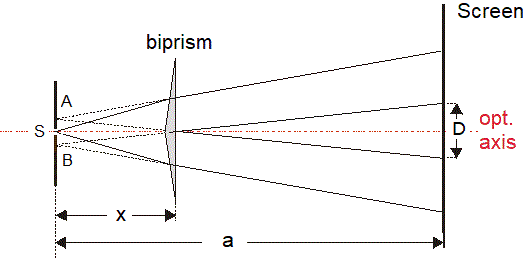Calculate the wavelength if monochromatic light is used from the quantities given in the sketch and the distance ##\Delta y## between two adjacent interference strips? Assume that the dimensions parallel to the optical axis can be viewed as large compared to those perpendicular to the optical axis.

14. (solved by @Not anonymous ) A galaxy is ##42\,\rm MLy## away and oriented in space, such that its rotation axis is perpendicular to the line of sight. The ##\alpha## line of hydrogen is measured to occur at ##\lambda_1=658.003\,\rm nm## instead of ##\lambda _0=656.28 \,\rm nm## widened to ##b=0.438\,\rm nm.##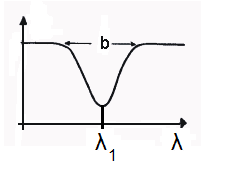Assume that the main cause of the widening is the rotation of the stars around the center of the galaxy. Assume further that the different wavelength is only due to the radial motion of the galaxy compared to our solar system.

What is the maximal rotational velocity of the observed stars, and what is the maximal velocity the galaxy is moving, and in which direction as seen from our solar system?

15. (solved by @Not anonymous ) The spiral galaxy ##\rm M81## near Ursa Major can already be viewed by a small telescope. It has an apparent magnitude of ##M=6.9.## The angle to the celestial pole is about ##21°.## Is it possible to observe ##\rm M81## the entire year, if you live in Toronto?

The following diagram shows data-points of light from the cepheid ##\rm C27## in ##\rm M81.##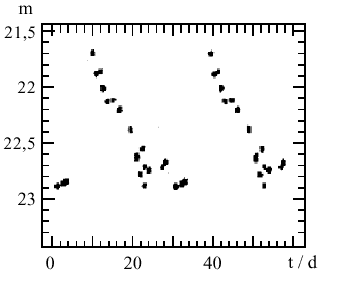Calculate our distance from ##\rm M81## in lightyears. (Use an average value of magnitude ##22.3## at a pulsation rate of ##30## per day and the relation ##M=-1.67-2.54\cdot\log_{10} p##.)

The second diagram is a comparison between ##\rm M81## and Milky Way. It shows the radial orbit velocity ##v## of the stars in relation to their distance ##r## from the galaxy center. Optical wavelengths are hardly to observe from around ##16\,kpc## on, so radio wavelengths are used.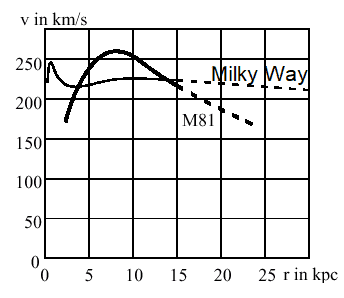Verify that if a celestial body orbits a center of great mass, then we can calculate the central mass approximately by ##M=\dfrac{v^2\cdot r}{G}.## Show by choosing two data-points that the rotation curve of ##\rm M81## is approximately ##v\sim \dfrac{1}{\sqrt{r}}## for ##r=10\,\rm{kpc}.## What does that mean for the mass distribution in ##\rm M81\,##? Estimate the mass of ##\rm M81## within the optical spectrum in units of sun masses.

The rotation curves of ##\rm M81## and the Milky Way differ a lot for great distances from the center. What does that mean for the mass distribution in our Milky Way?

The wavelength of the ##\alpha ##-line of hydrogen from the optical center of ##\rm M81## is measured to be ##\lambda_1=656.38\,\rm nm## in comparison to ##\lambda_0 =656.47 \,\rm nm.## Can we apply Hubble's law to ##\rm M81##?#

Last edited:
•Not anonymous and JD_PM

(3).

Clearly the set of algebraic numbers ##\mathbb{A}## is infinite, because it is contains the rational numbers. Indeed, if ##q = a/b## is a rational with ##a,b \in \mathbb{Z}## and ##b \ne 0##, then ##q## is a zero of ##Xb-a##.

Next, denoting the polynomials of degree ##n## and integer coefficients by ##\mathbb{Z}_n[X]##, observe that ##\mathbb{A}= \bigcup_{n=1}^\infty \bigcup_{P \in \mathbb{Z}_n[X]}\{x \in \mathbb{R}: P(x)=0\}##. Because a polynomial of degree ##n## has at most ##n## roots, we find
$$|\mathbb{A}| \le \sum_{n=1}^\infty \sum_{P \in \mathbb{Z}_n[X]} n = \sum_{n=1}^\infty n \aleph_0 = \sum_{n=1}^\infty \aleph_0 = \aleph_0$$ where it was implicitly used that ##|\mathbb{Z}[X]| = \aleph_0##. We conclude that ##|\mathbb{A}| = \aleph_0##, so ##\mathbb{A}## is countably infinite.

About question (1) I am a little bit confused. Isn't completeness invariant under changing to an equivalent metric? If so, why would you want to change to an equivalent metric?

julian
Gold Member
Problem #8

Let

\begin{align*}
S (z) & = \dfrac{az + b}{cz + d} \quad (ad -bc \not= ) \quad and \quad T (z) = \dfrac{a'z + b'}{c'z + d'} \quad (a'd' - b'c' \not= 0)
\end{align*}

\begin{align*}
S \circ T (z) & = S (T (z))
\nonumber \\
&= \dfrac{a \dfrac{a'z +b'}{c'z + d'} + b}{c \dfrac{a'z +b'}{c'z + d'} + d}
\nonumber \\
& = \dfrac{a (a'z +b') +b (c'z + d')}{c (a'z +b') + d (c'z + d')}
\nonumber \\
& = \dfrac{(aa' + bc') z + (ab' + bd')}{(ca' + dc') z + (cb' + dd')}
\end{align*}

There is an obvious relation to matrix multiplication:

\begin{align*}
\begin{pmatrix}
a & b \\
c & d
\end{pmatrix}
\begin{pmatrix}
a' & b' \\
c' & d'
\end{pmatrix}
=
\begin{pmatrix}
aa' + bc' & ab' + bd' \\
ca' + dc' & cb' + dd'
\end{pmatrix}
\end{align*}

Taking determinants

\begin{align*}
\det
\begin{pmatrix}
aa' + bc' & ab' + bd' \\
ca' + dc' & cb' + dd'
\end{pmatrix}
= \det
\begin{pmatrix}
a & b \\
c & d
\end{pmatrix}
\det
\begin{pmatrix}
a' & b' \\
c' & d'
\end{pmatrix}
\not= 0
\end{align*}

establishing that ##S \circ T (z)## is indeed a Mobius transformation, hence we have closure under composition. The relation to matrix multiplication establishes associativity under composition of Mobius transformations.

The identity corresponds to ##a = d \not= 0## and ##b = c = 0##:

\begin{align*}
\text{id} (z) & = z
\end{align*}

The relation to matrices implies the inverse is

\begin{align*}
S^{-1} (z) & = \dfrac{dz - b}{-cz + a} .
\end{align*}

So we have established that we have a group under composition of Mobius transformations.

Next part of the question: Having a ##(z - z_2)## in the numerator will mean ##S (z_2) = 0##. Having a ##(z - z_3)## in the denominator will mean ##S (z_3) = \infty##. So Consider

\begin{align*}
S (z) & = \dfrac{(z - z_2) A}{(z - z_3) B} .
\end{align*}

Then

\begin{align*}
S (z_1) & = \dfrac{(z_1 - z_2) A}{(z_1 - z_3) B} .
\end{align*}

So that if we choose ##A = (z_1 - z_3)## and ##B = (z_1 - z_2)## then ##S (z_1) = 1##. So finally, the Mobius transformation we are looking for is:

\begin{align*}
S (z) & = \dfrac{(z - z_2) (z_1 - z_3)}{(z - z_3) (z_1 - z_2)} .
\end{align*}

In this case

\begin{align*}
ad - bc & = (z_1 - z_3) [-z_3 (z_1 - z_2)] - [-z_2 (z_1 - z_3) (z_1 - z_2)]
\nonumber \\
& = (z_1 - z_3) (z_1 - z_2) (z_2 -z_3)
\end{align*}

this is not equal to zero as long as ##z_1,z_2,z_3## are distinct, which is required to be the case as a Mobius transformation is single valued.

Last edited:
•Delta2
Mentor
(3).

Clearly the set of algebraic numbers ##\mathbb{A}## is infinite, because it is contains the rational numbers. Indeed, if ##q = a/b## is a rational with ##a,b \in \mathbb{Z}## and ##b \ne 0##, then ##q## is a zero of ##Xb-a##.

Next, denoting the polynomials of degree ##n## and integer coefficients by ##\mathbb{Z}_n[X]##, observe that ##\mathbb{A}= \bigcup_{n=1}^\infty \bigcup_{P \in \mathbb{Z}_n[X]}\{x \in \mathbb{R}: P(x)=0\}##. Because a polynomial of degree ##n## has at most ##n## roots, we find
$$|\mathbb{A}| \le \sum_{n=1}^\infty \sum_{P \in \mathbb{Z}_n[X]} n = \sum_{n=1}^\infty n \aleph_0 = \sum_{n=1}^\infty \aleph_0 = \aleph_0$$ where it was implicitly used that ##|\mathbb{Z}[X]| = \aleph_0##. We conclude that ##|\mathbb{A}| = \aleph_0##, so ##\mathbb{A}## is countably infinite.
Well, the question was how to achieve an enumeration, i.e. why is ##\left|\mathbb{N}^\mathbb{N}\right|=\aleph_0=|\mathbb{Z}[X]|##? How can we be sure that there is no diagonalization argument possible between your inequalities?

Mentor
About question (1) I am a little bit confused. Isn't completeness invariant under changing to an equivalent metric? If so, why would you want to change to an equivalent metric?
And how do you show that ##(X,\rho)## is complete? The problem provides a criterion. The point is to change to a metric that is equivalent but provides more information.

Mentor
Problem #8

Let

\begin{align*}
S (z) & = \dfrac{az + b}{cz + d} \quad (ad -bc \not= ) \quad and \quad T (z) = \dfrac{a'z + b'}{c'z + d'} \quad (a'd' - b'c' \not= 0)
\end{align*}

\begin{align*}
S \circ T (z) & = S (T (z))
\nonumber \\
&= \dfrac{a \dfrac{a'z +b'}{c'z + d'} + b}{c \dfrac{a'z +b'}{c'z + d'} + d}
\nonumber \\
& = \dfrac{a (a'z +b') +b (c'z + d')}{c (a'z +b') + d (c'z + d')}
\nonumber \\
& = \dfrac{(aa' + bc') z + (ab' + bd')}{(ca' + dc') z + (cb' + dd')}
\end{align*}

There is an obvious relation to matrix multiplication:

\begin{align*}
\begin{pmatrix}
a & b \\
c & d
\end{pmatrix}
\begin{pmatrix}
a' & b' \\
c' & d'
\end{pmatrix}
=
\begin{pmatrix}
aa' + bc' & ab' + bd' \\
ca' + dc' & cb' + dd'
\end{pmatrix}
\end{align*}

Taking determinants

\begin{align*}
\det
\begin{pmatrix}
aa' + bc' & ab' + bd' \\
ca' + dc' & cb' + dd'
\end{pmatrix}
= \det
\begin{pmatrix}
a & b \\
c & d
\end{pmatrix}
\det
\begin{pmatrix}
a' & b' \\
c' & d'
\end{pmatrix}
\not= 0
\end{align*}

establishing that ##S \circ T (z)## is indeed a Mobius transformation, hence we have closure under composition. The relation to matrix multiplication establishes associativity under composition of Mobius transformations.

The identity corresponds to ##a = d \not= 0## and ##b = c = 0##:

\begin{align*}
\text{id} (z) & = z
\end{align*}

The relation to matrices implies the inverse is

\begin{align*}
S^{-1} (z) & = \dfrac{dz - b}{-cz + a} .
\end{align*}

So we have established that we have a group under composition of Mobius transformations.

Next part of the question: Having a ##(z - z_2)## in the numerator will mean ##S (z_2) = 0##. Having a ##(z - z_3)## in the denominator will mean ##S (z_3) = \infty##. So Consider

\begin{align*}
S (z) & = \dfrac{(z - z_2) A}{(z - z_3) B} .
\end{align*}

Then

\begin{align*}
S (z_1) & = \dfrac{(z_1 - z_2) A}{(z_1 - z_3) B} .
\end{align*}

So that if we choose ##A = (z_1 - z_3)## and ##B = (z_1 - z_2)## then ##S (z_1) = 1##. So finally, the Mobius transformation we are looking for is:

\begin{align*}
S (z) & = \dfrac{(z - z_2) (z_1 - z_3)}{(z - z_3) (z_1 - z_2)} .
\end{align*}

In this case

\begin{align*}
ad - bc & = (z_1 - z_3) [-z_3 (z_1 - z_2)] - [-z_2 (z_1 - z_3) (z_1 - z_2)]
\nonumber \\
& = (z_1 - z_3) (z_1 - z_2) (z_2 -z_3)
\end{align*}

this is not equal to zero as long as ##z_1,z_2,z_3## are distinct, which is required to be the case as a Mobius transformation is single valued.
Right. But why is it unique?

julian
Gold Member
Right. But why is it unique?

\begin{align*}
0 = S (z_2) = \dfrac{a z_2 + b}{c z_2 + d} \quad \text{implies} \quad z_2 = -b/a
\end{align*}

Hence ##z_2## is unique.

\begin{align*}
\infty = S (z_3) = \dfrac{a z_3 + b}{c z_3 + d} \quad \text{implies} \quad z_3 = -d/c
\end{align*}

Hence ##z_3## is unique. Finally,

\begin{align*}
\frac{c}{a} = \frac{c}{a} S (z_1) = \dfrac{z_1 - (- b/a)}{z_1 - (- d/c)} = \dfrac{z_1 - z_2}{z_1 - z_3}
\end{align*}

which rearranged gives

\begin{align*}
z_1 = \dfrac{z_3 \frac{c}{a} - z_2}{\frac{c}{a} - 1}
\end{align*}

meaning that ##z_1## is unique.

Mentor
\begin{align*}
0 = S (z_2) = \dfrac{a z_2 + b}{c z_2 + d} \quad \text{implies} \quad z_2 = -b/a
\end{align*}

Hence ##z_2## is unique.

\begin{align*}
\infty = S (z_3) = \dfrac{a z_3 + b}{c z_3 + d} \quad \text{implies} \quad z_3 = -d/c
\end{align*}

Hence ##z_3## is unique. Finally,

\begin{align*}
\frac{c}{a} = \frac{c}{a} S (z_1) = \dfrac{z_1 - (- b/a)}{z_1 - (- d/c)} = \dfrac{z_1 - z_2}{z_1 - z_3}
\end{align*}

which rearranged gives

\begin{align*}
z_1 = \dfrac{z_3 \frac{c}{a} - z_2}{\frac{c}{a} - 1}
\end{align*}

meaning that ##z_1## is unique.
The general argument is:

Let ##S\not\equiv 1## and ##S(z)=z.## Then ##0=cz^2+(d-a)z-b## which has at most two different solutions. If ##a,b,c\in \mathbb{C}_\infty ## are three different points such that ##S(a)=T(a),S(b)=T(b),S(c)=T(c)## for two Möbius transformations ##S,T.## Then ##T^{-1}\circ S## has three fixed points, i.e. ##T^{-1}S\equiv 1## and ##S=T.## Hence, a Möbius map is uniquely determined by its action on any three distinct given points in ##\mathbb{C}_\infty .##

•dextercioby and julian
Well, the question was how to achieve an enumeration, i.e. why is ##\left|\mathbb{N}^\mathbb{N}\right|=\aleph_0=|\mathbb{Z}[X]|##? How can we be sure that there is no diagonalization argument possible between your inequalities?

##|\mathbb{Z}[X]| = \aleph_0## is quite easy to prove. Indeed, ##\mathbb{Z}[X]= \bigcup_{n=0}^\infty \mathbb{Z}_n[X]## so since ##\mathbb{Z}_n[X] \cong \mathbb{Z}^{n+1} \cong \mathbb{N}##, we find $$|\mathbb{Z}[X]| \le \sum_{n=0}^\infty |\mathbb{Z}_n[X]| \le \sum_{n=0}^\infty \aleph_0 = \aleph_0.$$
I'm not sure what you mean by diagonalisation argument. All I did was applying standard cardinality arithmetic.

Btw, ##|\mathbb{N}^{\mathbb{N}}| = \aleph_0## is false, and I never used that.

Thanks for the feedback :)

Mentor
##|\mathbb{Z}[X]| = \aleph_0## is quite easy to prove. Indeed, ##\mathbb{Z}[X]= \bigcup_{n=0}^\infty \mathbb{Z}_n[X]## so since ##\mathbb{Z}_n[X] \cong \mathbb{Z}^{n+1} \cong \mathbb{N}##, we find $$|\mathbb{Z}[X]| \le \sum_{n=0}^\infty |\mathbb{Z}_n[X]| \le \sum_{n=0}^\infty \aleph_0 = \aleph_0.$$
I'm not sure what you mean by diagonalisation argument. All I did was applying standard cardinality arithmetic.
##|\mathbb{Z}^n|=\aleph_0## ok, but what is ##|\mathbb{Z}^\infty |## since you sum over all ##n##?

The "easy to prove" of all of your ##\leq## signs are what the problem is about. Find a smart enumeration, or do it the long way and prove the inequalities, especially the one with ##\sum^\infty ##.

##|\mathbb{Z}^n|=\aleph_0## ok, but what is ##|\mathbb{Z}^\infty |## since you sum over all ##n##?

The "easy to prove" of all of your ##\leq## signs are what the problem is about. Find a smart enumeration, or do it the long way and prove the inequalities, especially the one with ##\sum^\infty ##.

Are you telling me that I cannot use the standard cardinal arithmetic inequalities ##|\bigcup_{i \in I} A_i| \le \sum_{i \in I}|A_i| \le |I| \sup_{i \in I} |A_i|##? Because really that's all I'm using, together with the fact that ##\aleph_0 \aleph_0 = \aleph_0##. Cardinal arithmetic is invented to not have to find explicit bijections!

##|\mathbb{Z}^n|=\aleph_0## ok, but what is ##|\mathbb{Z}^\infty |## since you sum over all ##n##?

The "easy to prove" of all of your ##\leq## signs are what the problem is about. Find a smart enumeration, or do it the long way and prove the inequalities, especially the one with ##\sum^\infty ##.

Also, you can here appeal to the fact that countable union of countable sets is countable, if you are ok with ##|\mathbb{Z}_n[X]| = \aleph_0##. Maybe you are right and I overcomplicate the issue.

Mentor
In a way, yes, that's what I was saying. Cardinal arithmetic is nothing many readers use very often, so it would've been nice to show why it can be used, or why it is correct. E.g.

##|\bigcup_{i \in I} A_i| \le \sum_{i \in I}|A_i| \le \underbrace{|I|}_{=\aleph_0} \underbrace{\sup_{i \in I} |A_i|}_{=\aleph_0}##

could have been explained by the fact that this is the cardinality of ##\mathbb{Q}## and as such denumerable. And an explicit enumeration would have been quite as easy to find. The point was to see, that although we have a considerable large extension of ##\mathbb{Q}##, we are still on the countable side.

Edit: Otherwise an answer "Obvious." would have been sufficient, too.

Attempt for an elementary solution for (3):

Alright, so let me try to give a more elementary approach then. All I will use is that the countable union of countable sets is again countable, which is proven using a standard diagonalisation argument.

We have ##\mathbb{Z}[X]= \bigcup_{n=0}^\infty \mathbb{Z}_n[X]##, so ##\mathbb{Z}[X]## is countable, as a countable union of countable sets.

Given a polynomial ##P\in \mathbb{Z}[X]##, let ##V(P)## be the set of its zeros. Note that ##V(P)## is a finite set. If ##\mathbb{A}## is the set of algebraic integers, we find
$$\mathbb{A}= \bigcup_{P \in \mathbb{Z}[X]} V(P)$$
which is again countable, as a countable union of countable sets.

Mentor
Attempt for an elementary solution for (3):

Alright, so let me try to give a more elementary approach then. All I will use is that the countable union of countable sets is again countable, which is proven using a standard diagonalisation argument.

We have ##\mathbb{Z}[X]= \bigcup_{n=0}^\infty \mathbb{Z}_n[X]##, so ##\mathbb{Z}[X]## is countable, as a countable union of countable sets.

Given a polynomial ##P\in \mathbb{Z}[X]##, let ##V(P)## be the set of its zeros. Note that ##V(P)## is a finite set. If ##\mathbb{A}## is the set of algebraic integers, we find
$$\mathbb{A}= \bigcup_{P \in \mathbb{Z}[X]} V(P)$$
which is again countable, as a countable union of countable sets.
It's ok. It is really not that difficult, which makes it harder to judge what between
"Obvious"
and
"Set ##w(f)=\deg f + \operatorname{card}\{x\,|\,f(x)=0\}## and order it e.g. lexicographically."
is a solution and what is not.

6. Let Fq be a finite field of characteristic p. Show that it's multiplicative group Fq∗=Fq−{0} is cyclic.
Let me try NO.6, to find out what have left to me after the abstract algebra course.

Because, those q-1 nonzero elements of ##F_q## are roots of x^(q-1)-1, which is the polynomial over F_q, and a simple lemma, that is, the number of roots of a polynomial over field is less than the degree of the polynomial, we know that x^(q-1)-1 is equal to the mutiple of all the x-##\alpha##, where the ##\alpha## is a nonzero element of ##F_q##. So, if the nonzero element who get the biggest order in the multiplicative group, still, whose order is smaller than q-1, which means those q-1 nonzero elements are roots of a polynomial ##x^n-1##, where n|(q-1). The last conclusion need a lemma to show that for the communicative group, all the order divides the biggest order, which can be proved by considering a*b, where a,b are two elements whose orders cannot divide (the inverse is divided) each other. That is a contradiction, ##x^n-1## has more than n roots.

Mentor
I cannot give you a counterexample to show where you go wrong, since the statements are true. But I do not see where you get the single generator. Let's pretend we had ##V_4## as group. Then all your arguments apply but ##V_4## isn't cyclic.

As far as I can see you only use
$$\mathbb{F_q}^*=\{x\,:\,x^{q-1}=1\}=\{\alpha \,:\,(X-\alpha )\,|\,(X^{q-1}-1)\}=\{x\,:\,\operatorname{ord}(x)\,|\,(q-1)\}$$
which is true, but how is it cyclic? You need something of the form ##A_1\wedge A_2\wedge \ldots \wedge A_n \Longrightarrow G=\langle x \rangle.##

Sorry, what is ##V_4##?
I think I prove that the element which get the biggest order is the single generator, using the lemma that the biggest order (assume that is n) can be divided by any other order (any other element's order), here is the lemma:
The last conclusion need a lemma to show that for the communicative group, all the order divides the biggest order, which can be proved by considering a*b, where a,b are two elements whose orders cannot divide (the inverse is divided) each other.

Then ##x^n-1## has all the elements as its roots, which is a contrary to n<q-1. But, yes, I shouldn't mention x^(q-1)-1=all the ##x-\alpha## multiply at the begining of my answer, it is irrelevant to the question.

Mentor
Just because the order divides the order of another element, does not make them powers of each other. ##V_4=\langle a,b\,|\,a^2,b^2,(ab)^2 \rangle## is the Klein four-group.

PeroK
Homework Helper
Gold Member
2020 Award
For question 11, why doesn't the gardener tilt the hose up at an angle? It's better than holding the hose above his head, surely?

Just because the order divides the order of another element, does not make them powers of each other. ##V_4=\langle a,b\,|\,a^2,b^2,(ab)^2 \rangle## is the Klein four-group.
What I proved is: all the elements of ##F_q*## is a root of x^n-1, and n is the biggest order.
Then x^n−1 has all the elements as its roots, which is a contrary to n<q-1

I think, to prove "make them the powers of each other", I must wait until having proved the F_q* is cylic group.
For Klein group, true it is communicative, but it is not a ring, then we cannot say the polynomial over ##V_4##. but for the field ##F_q##, we can say the ##F_q[x]## is UFD (Unique Factorization Domain), since everybody knows F[x] is UFD (where F is a field), by using the division with remainder.
Btw, using UFD can get the conclusion that "the number of roots is less than the degree, for f(x)".

Mentor
What I proved is: all the elements of ##F_q*## is a root of x^n-1, and n is the biggest order.

I think, to prove "make them the powers of each other", I must wait until having proved the F_q* is cylic group.
For Klein group, true it is communicative, but it is not a ring, then we cannot say the polynomial over ##V_4##. but for the field ##F_q##, we can say the ##F_q[x]## is UFD (Unique Factorization Domain), since everybody knows F[x] is UFD (where F is a field), by using the division with remainder.
Btw, using UFD can get the conclusion that "the number of roots is less than the degree, for f(x)".
There is nothing to add to what I already have said in posts #18 and #20. I know that ##V_4\ncong \mathbb{F}_5^*## because the latter is cyclic. However, your proof doesn't distinguish between the two.

For every element ##x\in G## of a finite group ##G## with ##n## elements holds ##x^n=1##. Therefore ##X^{q-1}-1=0## has exactly the ##n=q-1## roots of ##\mathbb{F}_q^*.##

And why is there a primitive root?

For every element x∈G of a finite group G with n elements holds xn=1. Therefore Xq−1−1=0 has exactly the n=q−1 roots of Fq∗.
Omg. In group, we have only one operation, like you called it, "*". We don't have "+".
I think maybe you have another way to prove it, but you truly didn't understand my way (whether it is true or not), and I say UFD is a critical thing, so is that I do it too sophisticated?
Still, sorry for my poor English and poor LaTeX, which may contribute to the problem in sending my message.

11. A gardener holds a water hose horizontally and wants to water a bed ##6 \ \text{m}## away. The water exits the hose at a speed of ##8 \ \text{m/s}##. Calculate the minimum height the gardener needs to hold the hose for the water to reach the bed, the speed at which the water droplets hit the bed, and the angle at which the water droplets hit the bed.

In the attempted solution, I assume that the effect of air resistance or friction on the water jet can be ignored.

Let ##h## be the height at which the hose is held and ##T## be the time taken by a water drop to touch the ground after exiting the hose. The horizontal distance covered by water before reaching the ground is ##8T \ \text{m}##. Since ##T## increases with the vertical distance ##h## to be covered, the horizontal distance covered too increases with increasing height. The minimum height needed to ensure that the water reaches the garden bed is the value for which ##8T = 6 \Rightarrow T = 0.75##, i.e. time of vertical fall just enough to allow water to cover the desired horizontal distance too.

##h##, the vertical distance covered by free fall till touching the ground, is given by $$h = \int_{t=0}^T v\, dt = \int_{t=0}^T gt\, dt = \frac{gT^{2}} {2} \Rightarrow h = \frac{9.8 \times 0.75^{2}} {2} = 2.75625 \, \text{m}$$

In the above equation, ##v## denotes the vertical velocity at instant ##t##, and ##g## is the gravitational acceleration at earth's surface level.

The vertical velocity of water at the instant of hitting the ground is $$V_{vertical} = \int_{t=0}^T g\, dt = gT = 9.8 \times 0.75 = 7.35 \, \text{m/s}$$

The speed ##S## at which the water hits the garden bed equals water stream's velocity along the direction at which it hits the ground and is given by $$S = \sqrt{V_{vertical}^2 + V_{horizontal}^2} = \sqrt{7.35^2 + 8^2} ≈ 10.864\, \text{m/s}$$

The angle at which water hits the garden bed is given by $$θ = \tan^{-1} \frac{V_{vertical}} {V_{horizontal}} = \tan^{-1} \frac{7.35} {8} ≈ 42.575°$$

•fresh_42